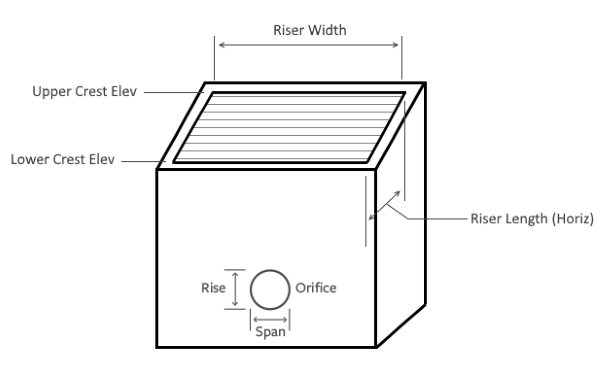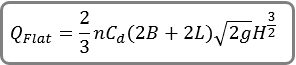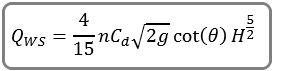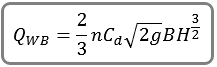# Sloped Risers

Sloped Risers are a special type of riser box that are rectangular in shape and can have unique elevations at the top and bottom crests. The methodology follows what is recommended in the U.S. Bureau of Reclamation Technical Memorandum PAP-1105, “Physical Modeling of Overflow Outlets for Extended Detention Stormwater Basins”.

It models unsubmerged, submerged as well as inlet control (orifice) conditions. It includes coefficients that take into account the net, clear opening area of a grate as well as clogging. These coefficients are:

Cd = Discharge coefficient (typically 0.62)
n = Open area ratio for the grate (typically between 0.3 and 0.7)Sloped Riser shown with a multi-stage orifice

## Flat Riser

The sloped riser, although meant to have a sloped top to match the side slope of a detention pond, may be completely flat. In those cases, the following equation is used:Where:

QFlat = total discharge (cfs)
B = inside riser width (ft)
L = inside riser length (ft)
g = gravity
H = depth of water above the lower crest elevation

## Sloped Unsubmerged

The following equations are used when the water surface is between the lower and upper crest elevations.Where:

QWS = discharge over the side (cfs)
QWB = discharge over the lower front of the riser
θ = Angle of the riser top slope from the horizontal
g = gravity
H = depth of water above the lower crest elevation (ft)
B = inside riser width (ft)

Total discharge = 2QWS + QWB

## Sloped Submerged

This equation is used when the water surface is above the upper crest elevation:Where:
QWS = discharge over the side (cfs)
H = depth of water above the lower crest elevation (ft)
Hb = upper crest elevation – lower crest elevation (ft)

Total discharge = QWB + 2QWS + QWT

QWT uses the same equation as QWB where H = depth of water above the upper crest elevation.